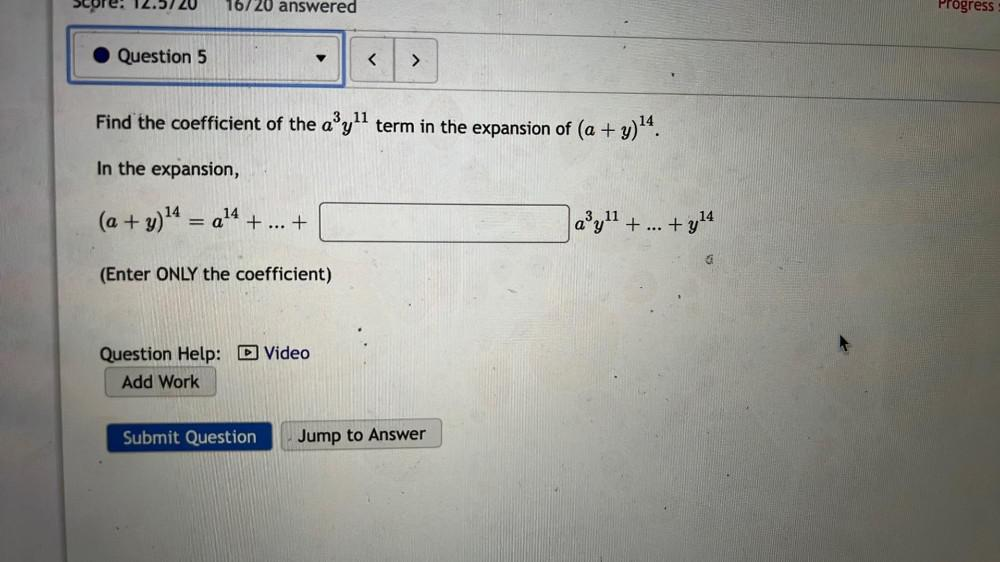Question:

# Find the coefficient of the a³y¹¹ term in the expansion of (a + y)¹⁴. In the expansion, (a + y)¹⁴ = a¹⁴ + ... +___________ a³y¹¹ + ... +y¹⁴ (Enter ONLY the coefficient)Find the coefficient of the a³y¹¹ term in the expansion of (a + y)¹⁴. In the expansion, (a + y)¹⁴ = a¹⁴ + ... +___________ a³y¹¹ + ... +y¹⁴ (Enter ONLY the coefficient)